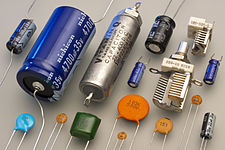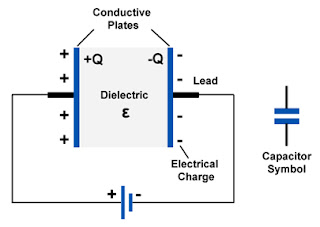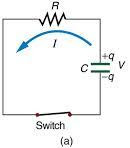# Capacitor - an IntroductionThe ability to store energy in the form of electric charge is called capacitance. A device designed to possess capacitance is called a capacitor. A capacitor is a two terminal device used to store energy in the form of electric field. The symbol used to represent capacitance is C. A  capacitor in its simplest form a capacitor is nothing more than two conducting plates separated by a sheet of insulator. In capacitors the conductors are called plates and the insulator is called a dielectric. The larger the surface area of the "plates" (conductors) and the narrower the gap between them, the greater the capacitance is.

### Basic Capacitor ActionA capacitor stores energy when an electric charge is forced onto its plates by some other energy source, such as a battery. When there is a potential difference across the terminals, i.e. when the capacitor is connected across a battery as shown in figure below, an electric field develops across the dielectric, causing positive charge +Q to collect on one plate and negative charge −Q to collect on the other plate. These charges on the plates of the capacitor represent a voltage source just as the charges on the plates of a cell do. Note that in the process of charging the capacitor, no electrons move from one plate to the other plate through the dielectric.Because of opposing voltages, the current stops once the capacitor is charged. The opposite charges on the plates of the capacitor create a new energy source. The energy stored in the capacitor produces a voltage equal to that of the battery. Since the capacitors voltage is equal to, and in opposition to, the battery voltage , a state of equilibrium exists. No current can flow in either direction.

A charged capacitor can be disconnected from the original energy- source (the battery and used as a new energy source. If a voltmeter is connected to the capacitor, it will register a voltage., if a resistor is connected to the capacitor as shown in figure current will flow through the resistor. However, a capacitor has limited use as a primary energy source for two reasons:
1. For its weight and size, the amount of energy it can store is small compared with what a battery can store.
2. The voltage available from the capacitor rapidly diminishes as energy is removed from the capacitor
Although the amount of energy stored in a capacitor is small, capacitor can deliver a shock. The shock can be very severe (even fatal) if the capacitor is large and charged to a high voltage.### Capacitance

Capacitance of a capacitor is defined as the ratio of charge Q on each conductor to the voltage V between them.
C = Q / V

### Unit of Capacitance

The base unit of capacitance is the Farad. The abbreviation for farad is F. One farad is that amount of capacitance which stores 1 C of charge when the capacitor is charged to 1V . In other words, a farad is a coulomb per volt  (C / V).

Example:
Question: What is the capacitance of a capacitor that requires 0.5 C to charge it to 25 V?

Given :           Charge (Q) = 0.5 C
Voltage (V) = 25V
Solution :       C = Q/V = 0.5/25 = 0.02

Answer :        The capacitance is 0.02 F.

The 0.02 F calculated above is a very high value of capacitance. In most circuits the capacitance used is much lower. In fact, they are usually so much lower that the base unit of farad is too high to conveniently express their value. The micro-farad (10-6) and the picofarad (pF)(10-12) are more convenient units. Both are used extensively in specifying values of capacitors in electronic circuits. Often it is necessary to convert from one unit to another.
1000 pF = 1000 x 10-6mF = 0.001 mF
0.001 mF = 0.001 x 106 pF     = 1000 pF

This post first appeared on Electrical Engineering Tutorial, please read the originial post: here

# Share the post

Capacitor - an Introduction

×

# Subscribe to Electrical Engineering Tutorial

Get updates delivered right to your inbox!

×